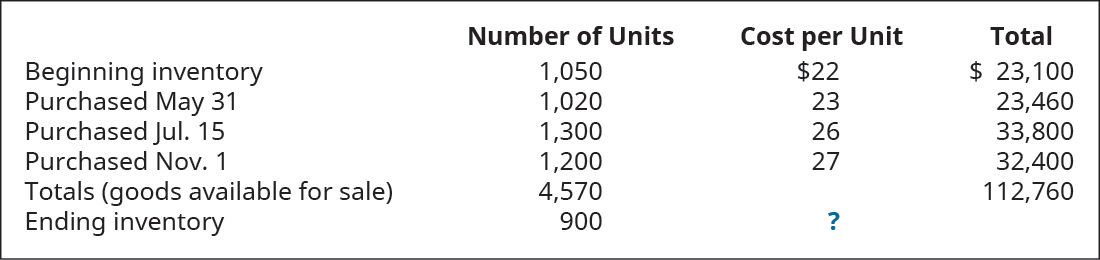# Trini Company had the following transactions for the month. Calculate the cost of goods sold dollar value for the period for each of the following cost allocation methods, using periodic inventory updating. Provide your calculations. A. first-in, first-out (FIFO) B. last-in, first-out (LIFO) C. weighted average (AVG)FindFindarrow_forward

### Principles of Accounting Volume 1

19th Edition
OpenStax
Publisher: OpenStax College
ISBN: 9781947172685

#### Solutions

Chapter
SectionFindFindarrow_forward

### Principles of Accounting Volume 1

19th Edition
OpenStax
Publisher: OpenStax College
ISBN: 9781947172685
Chapter 10, Problem 3PA
Textbook Problem
36 views

## Trini Company had the following transactions for the month.Calculate the cost of goods sold dollar value for the period for each of the following cost allocation methods, using periodic inventory updating. Provide your calculations.A. first-in, first-out (FIFO)B. last-in, first-out (LIFO)C. weighted average (AVG)

To determine

(a)

To compute:

Cost of Goods Sold for the period by using FIFO method.

Introduction:

First In First Out is a method of evaluation of cost of goods sold. In this method, it is assumed that units purchased at first is sold first. Costs in relation with units sold are taken from cost of first lot and in case, the sold units is higher than the first lot purchased, then cost of second lot is used.

### Explanation of Solution

Computation of COGS:

 Particulars Amount ($) Amount ($) Number of units sold (4,750−900) 3,670 Cost of Goods Sold: Opening: (1,050 units×22) 23,100 Add
To determine

(b)

To compute:

Cost of Goods Sold for the period by using LIFO method.

Introduction:

Last In First Out is a method of evaluation of cost of goods sold. In this method, it is assumed that units purchased at last is sold first. Costs in relation with units sold are taken from cost of last lot and in case, the sold units is higher than the latest lot purchased, then cost of last second lot is used.

To determine

(c)

To compute:

Cost of Goods Sold for the period by using Weighted Average Method.

Introduction:

Weighted Average Method is used to calculated cost of goods sold. In this method, cost allocated to sold units is computed by taking weighted average cost of the goods purchased.

### Still sussing out bartleby?

Check out a sample textbook solution.

See a sample solution

#### The Solution to Your Study Problems

Bartleby provides explanations to thousands of textbook problems written by our experts, many with advanced degrees!

Get Started

Find more solutions based on key concepts
Why must employers maintain employees individual earnings records?

College Accounting (Book Only): A Career Approach

Why do economists make assumptions?

Principles of Economics (MindTap Course List)

In Chapter 7, we saw that if the market interest rate, rd, for a given bond increased, the price of the bond wo...

Fundamentals of Financial Management, Concise Edition (with Thomson ONE - Business School Edition, 1 term (6 months) Printed Access Card) (MindTap Course List)

What is human capital management (HCM)?

Pkg Acc Infor Systems MS VISIO CD